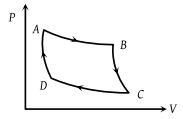# NEET Physics Thermodynamics Questions Solved

Carnot cycle (reversible) of a gas represented by a Pressure-Volume curve is shown in the diagram . Consider the following statements

I. Area ABCD = Work done on the gas

II. Area ABCD = Net heat absorbed

III. Change in the internal energy in cycle = 0

Which of these are correct ?(1) I only

(2) II only

(3) II and III

(4) I, II and III

(3) Work done by the gas (as cyclic process is clockwise) ∴ ΔW = Area ABCD

So from the first law of thermodynamics ΔQ (net heat absorbed) = ΔW = Area ABCD

As change in internal energy in cycle ΔU = 0.

Difficulty Level:

• 8%
• 14%
• 35%
• 45%
Crack NEET with Online Course - Free Trial (Offer Valid Till August 28, 2019)